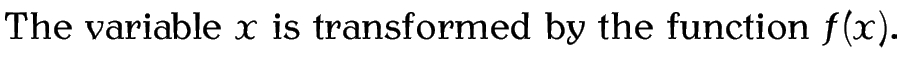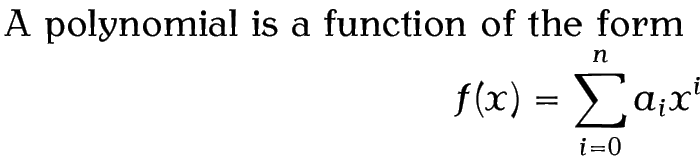9. Mathematics

As mentioned in the introduction, LaTeX is particularly good at typesetting mathematics. In order to use any of the maths commands we need to be in one of the mathematics environments. There are two basic types of mathematics: in-line maths and displayed maths. In-line maths is mathematics that occurs within a line of text, for example:Displayed maths is mathematics that occurs on a line of its own. For example:The maths environments switch to LaTeX's “math mode”, which uses specialist maths fonts and spacing rather than just using an italic font.

If you want to typeset any mathematics, I strongly advise using the amsmath package:

\usepackage{amsmath}
This patches some existing LaTeX commands and environments and also provides many useful additions.

This chapter is just an introduction to typesetting mathematics in LaTeX. If you want a comprehensive guide, I recommend you read Math Mode by Herbert Voß , which can be access via texdoc (see §1.1. Class and Package Documentation):

texdoc mathmode

This book is also available as A4 PDF or 12.8cm x 9.6cm PDF or paperback (ISBN 978-1-909440-00-5).

© 2012 Dickimaw Books. "Dickimaw", "Dickimaw Books" and the Dickimaw parrot logo are trademarks. The Dickimaw parrot was painted by Magdalene Pritchett.